### Researchers realize entanglement-enhanced quantum metrology in colored noise

2022-08-25

From the physics point of view, measurement is a physical process that estimates the quantity of a particular observable or a physical parameter. The science of measurement is called metrology. How to improve the precision in metrology is a central issue in this field, for which many classical approaches have been proposed.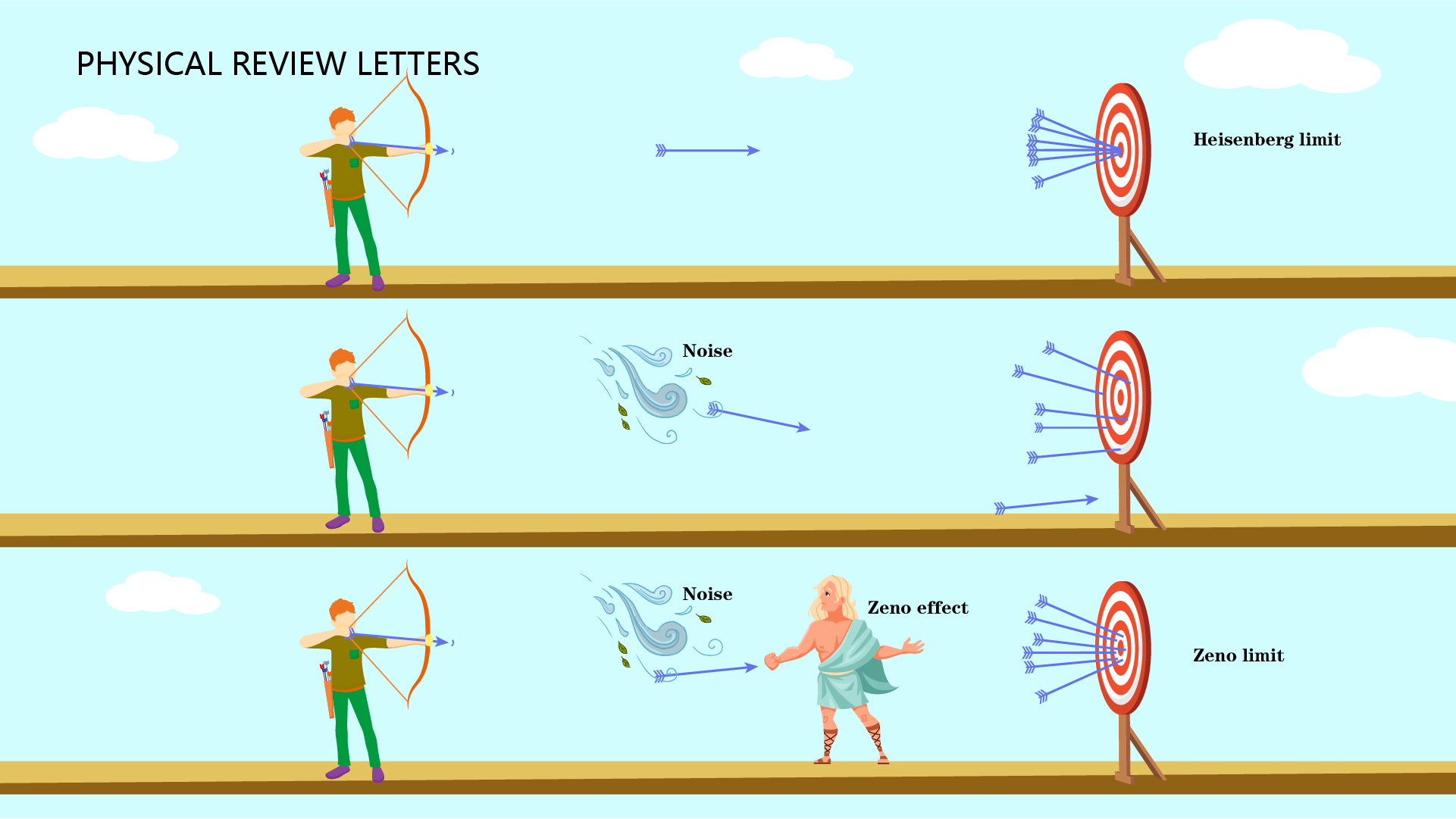Associate Professor Dawei Lu’s group from the Shenzhen Institute for Quantum Science and Engineering (SIQSE) and the Department of Physics at the Southern University of Science and Technology (SUSTech) has recently reported the experimental realization of entanglement-enhanced quantum metrology enhanced in colored noise.

Their research, entitled “Entanglement-Enhanced Quantum Metrology in Colored Noise by Quantum Zeno Effect,” has been published in Physical Review Letters, one of the most prestigious journals in physics.

Consider the example of measuring the thickness of a coin. A traditional way is to directly measure the thickness of a coin in a single experiment and repeat the measurement times. Assuming that the expectation of the measurement is exactly the real thickness of the coin, i.e., an unbiased estimate, the standard deviation of the final measured thickness will be proportional to N-1/2. One can use the modified approach to improve the precision by measuring the thickness of N coins in each run. After repeating the measurement by N times, the standard deviation of the physical quantity will be proportional to N-1. This reduces the measurement uncertainty by N-1/2 compared to the traditional method.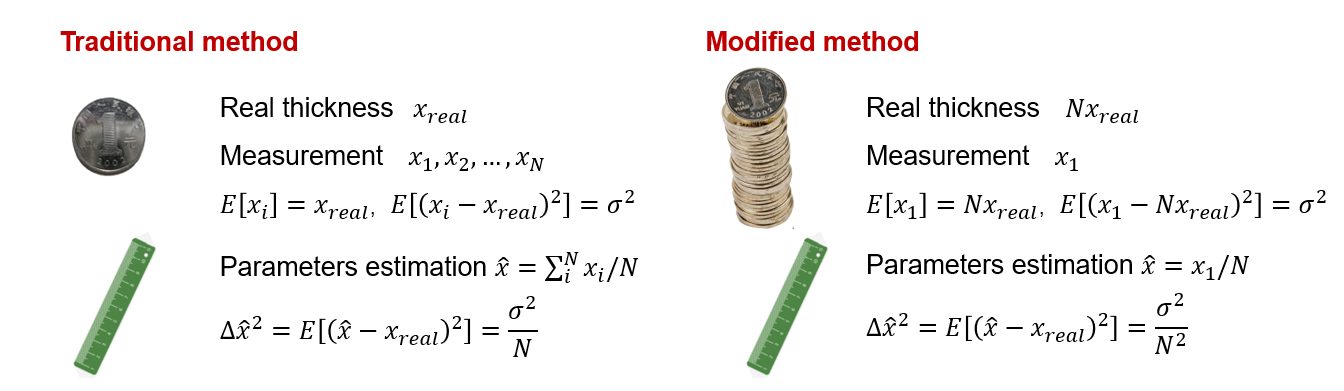Figure 1. Traditional and modified methods of measuring a coin’s thickness.

Prof. Lu’s group then considered a similar case in the quantum realm. In Ramsey interference experiments, if one wants to measure the energy splitting ω0 of a system Hamiltonian  H=ω0σz/2, a typical method consists of the following three steps: (i) preparing the initial state, (ii) evolving the state under the Hamiltonian and accumulating an energy-splitting dependent phase, and (iii) measuring the population p=[1-cos(ω0t)]/2.

In the traditional method, the input is a superposition state, so repeating the measurement by N times leads to the measurement uncertainty proportional to n-1/2. This is the well-known standard quantum limit (SQL) in quantum metrology. To improve the precision, one can use the maximally entangled state of n qubits as the input, by which the precision can reach the Heisenberg limit that scales as n-1. This is also the upper bound of precision due to the limitation of the Heisenberg uncertainty principle in quantum mechanics, which is usually achieved with the resources of maximally quantum entangled states (e.g., spin-compressed, NOON, GHZ, and Dicke states).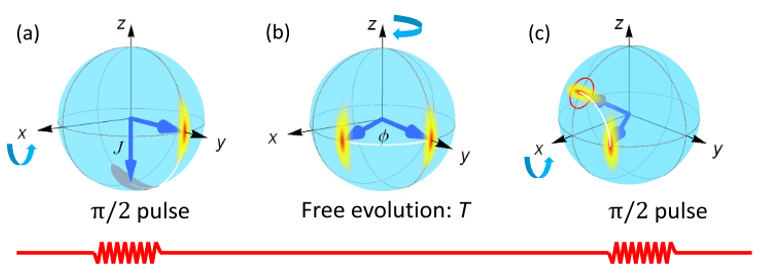Figure 2. Ramsey interference experiment.

However, the Heisenberg limit is the ideal case in quantum metrology. In reality, any quantum system unavoidably interacts with the surroundings, which leads to noise that prevents the metrology precision from achieving the Heisenberg limit. Depending on whether the environment of the quantum system provides memory that retains correlations across system-environment interactions, the noise can be classified into the Markovian and non-Markovian noise mechanisms.

A Markovian environment does not have a memory effect, which means that the state in the environment is not correlated, while a non-Markovian environment has an infinitely long memory. Typically, a physical environment in nature always owns the non-Markovian characteristic to some extent. Neither of the two noise mechanisms enables the reach of the Heisenberg limit with maximally quantum entangled states as probes.

In 2012, Chin, Huelga, and Plenio [PRL 109, 233601 (2012)] theoretically proved that the SQL could be surpassed with entangled probes in non-Markovian environments, which reached n-3/4. This is called the quantum Zeno effect (QZE) limit, in which the key idea is that QZE can significantly reduce the decoherence error.

This work is a milestone in the community of quantum metrology with over 500 citations, but the theory still awaits experimental demonstration. There are two main challenges to demonstrating Chin et al.’s theory. Firstly, although the QZE is widely present in various quantum systems, its application in quantum metrology requires pure non-Markovian environments, which is hard to realize in an experiment. Secondly, to show the scaling behavior of n-3/4 requires a relatively large number of qubits, which further increases the experimental difficulty.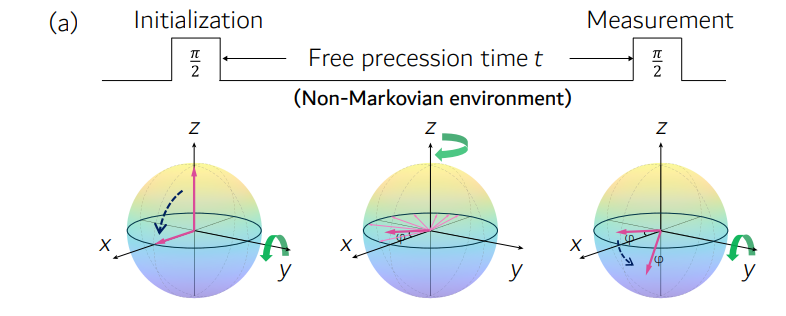Figure 3. Bath-engineering technique to simulate a high-precision non-Markovian environment.

To solve these two issues, Prof. Lu’s team modulated the noise mechanism by a quantum simulation approach, applying a bath-engineering technique to mimic a non-Markovian environment with high precision. Similar to the standard Ramsey interferometry experiment, the authors first prepared the initial state to the superposition state and then let it evolve under the time-modulated HamiltonianHSB (t)= ω0σz /2 +βz (t)σz, where βz (t) is some random error. The core of bath-engineering is to prepare a large number of identical initial states and let them evolve under different Hamiltonians for a period of time. Since each initial state experiences a different precession frequency, they eventually accumulate different phases after the evolution. By averaging the final states in ensemble (time), a purely decoherence (non-Markovian) environment can be simulated.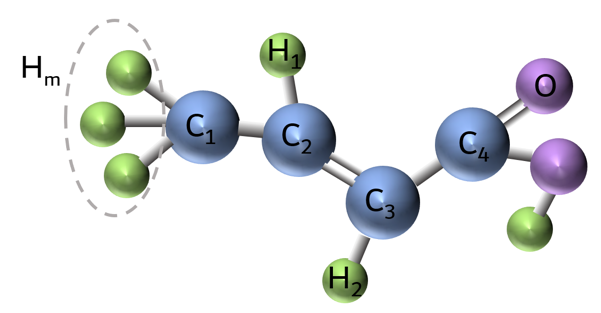Figure 4. Trans-crotonic acid (7-bit quantum simulator).

The researchers performed experiments on a nuclear magnetic resonance quantum simulator where the environmental modulation was achieved by using up to seven qubits. In association with the high-fidelity initialization of entangled probes and readout, it can be observed that the metrology precision of the target magnetic field is exactly enhanced by a factor of n1/4 using the entangled probe, which is exactly the QZE limit. In addition, as a reference, the Heisenberg limit is also demonstrated in another group of experiments without noise, thus setting up a complete set of experiments toward the entanglement-enhanced quantum metrology.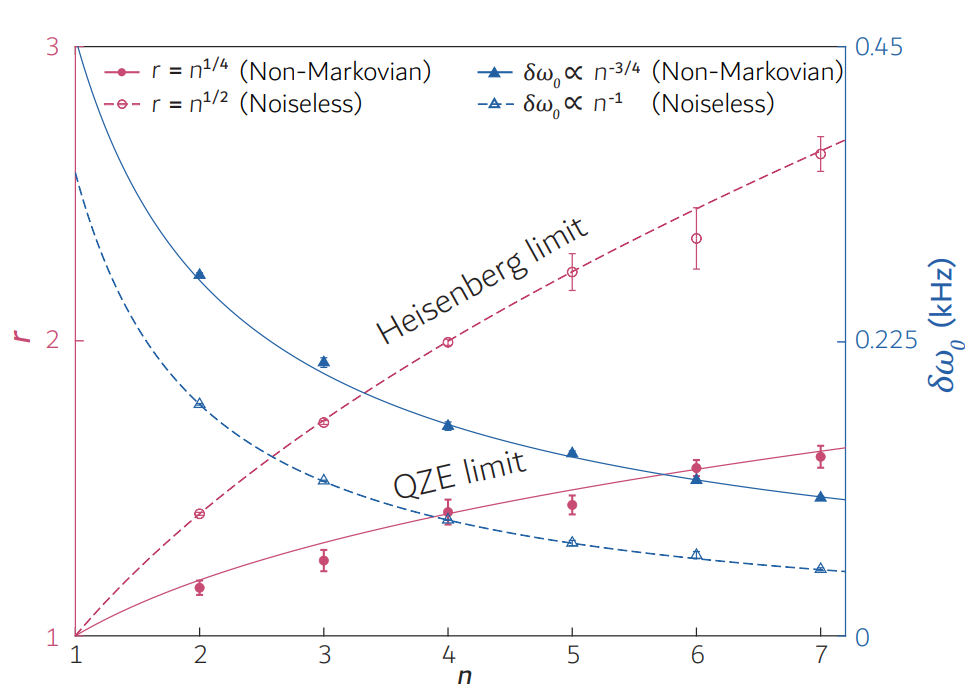Figure 5. Experimental results in non-Markovian noise and noiseless environments. Using the entanglement probe, the measurement precision under non-Markovian noise (solid red) achieves an enhancement of n1/4 with the number of qubits n. In contrast, the enhancement reaches n1/2 in the noiseless environment (dashed red), which is the Heisenberg limit.

In this work, the researchers applied a bath-engineering technique that does not require auxiliary qubits. It only requires collective single-qubit rotations about the z-axis, making extending to other physical systems easy. This experiment not only demonstrates Chin et al.’s theory but also provides a tool to implement non-Markovian dynamics in other quantum systems, thus paving the way for future exploration of quantum metrology in noisy environments.

This study was a collaborative effort between Assoc. Prof. Dawei Lu’s group from SUSTech and Assoc. Prof. Qing Ai’s team from Beijing Normal University (BNU).

Xingyue Long, a Ph.D. student from the Department of Physics at SUSTech, Wanting He, a Ph.D. student from the BNU, and Nana Zhang, a Lecturer from the Chongqing University of Posts and Telecommunications (CQUPT), are the co-first authors of this paper. Other co-authors include Jun Li and Tao Xin from SIQSE at SUSTech.

This research was supported by the Ministry of Science and Technology (MOST), National Natural Science Foundation of China (NSFC), Department of Science and Technology of Guangdong Province, Shenzhen Science and Technology Innovation Commission, Beijing Natural Science Foundation, SUSTech, and BNU.